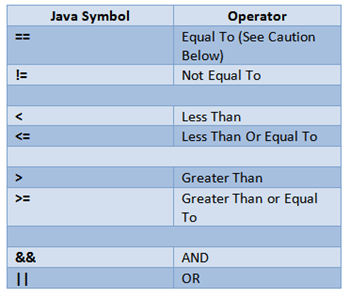# Java: Relational Operators

Instructor: Martin Gibbs

Martin has 16 years experience in Human Resources Information Systems, has a PhD in Information Technology Management, and a degree in Information Systems Management. He is an adjunct professor of computer science and computer programming.

Programming is all about testing and comparing values. Are they equal, or less than, or more than? The Java relational operators help us in these operations. This lesson will explain the operators and provide syntax examples. Updated: 05/25/2020

## Relational Operators

Can it really be true? Does the employee really have a pay rate higher than the allowed value? Did they have either sick pay or vacation this pay period?

The Java relational operators help us to ask questions. In technical speak, they help us check if a condition is met; but really they answer questions. Did the process complete successfully? Are the two values equal? If the answers to these questions is YES, Java considers them to be TRUE, and the condition is met.

In order to ask these questions, we need operators to tell Java how to answer them. The list of relational operators is shown in the following chart.Now let's look at each one and how they would work in Java code.

## Equal To (==)

Equal to is probably the most common operator. We are constantly checking to see if one value equals another value. The following code shows a code snippet that checks to see if the number entered is 15:

`if(enterMe == 15) { //value equals!`

Be very careful with the equal sign! If you are testing to see if a value is equal to another value, you MUST use both equal signs together (==). Not using these correctly can result in an assignment error. This means instead of comparing the values, you are actually ASSIGNING a value!

Note the error in this code. Thankfully the developer tool used displayed an error. Otherwise, the program would have assigned the value instead of comparing. This results in huge errors or programs that run out of control and crash.

`int i = 7;if(i=8) {  //code}`

## Not Equal To

Not equal to is another common question we ask in Java: What if the values aren't equal? Maybe we do some processing until they are or stop processing when they do not equal.

The following code shows a snippet of a loop that will process while the pay rate does not equal 15.

`float payRate;while(payRate != 15) { //code here}`

### Less Than/Greater Than

This operator also include conditions to test less-than-or-equal and greater-than-or-equal. The following code examples show a test for less than and greater than or equal to.

The following code will run as long as the variable maxTurns is less than 50:

`int maxTurns;do {  //game processing herewhile (maxTurns < 50);`

This code checks to see if the pay rate is greater than or equal to 15:

`if(payRate >= 15) {  //check state code}`

## Boolean Operators

Boolean operators are those that come out to either true or false. Technically, all of these operators are testing to see if a certain condition is TRUE. For example, if the order ID is less than 100, then the condition is considered true.

However, the AND and the OR statements in Java are very powerful because they let you combine the questions you are asking: Is the order ID less than 100 AND the customer ID greater than 50? Did the user enter either a 7 OR a 3?

Let's look at AND first. The following code checks to see if an employee pay rate is greater than 10 AND less than 20.

Notice how we combine other relational operators in these statements; this is the power of the Boolean operators and how they let us combine our questions.

`float payRate;if(payRate > 10 && payRate < 20) {  //pay rate is in the range} else {  // pay rate out of range}`

What if we are testing an employee to see which state they live in? Let's say we have laws for California and Maryland, and there are different laws for coding pay and other benefits. The following code checks to see if either of these conditions are true:

To unlock this lesson you must be a Study.com Member.
Create your account

### Register to view this lesson

Are you a student or a teacher?

### Unlock Your Education

#### See for yourself why 30 million people use Study.com

##### Become a Study.com member and start learning now.
Back
What teachers are saying about Study.com
Create an account to start this course today
Used by over 30 million students worldwide